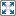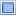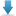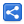# Java Regex - Capturing Group Reference in Replacement String

[Last Updated: Jul 15, 2017]

The capturing groups can be referenced in the matcher's replacement string.

#### Syntax:

1. `\${groupName}`: Using capturing group name 'groupName'. We must have defined the group name in the regex.
2. `\$groupNumber`: if we want to use group number instead of group name. May be we don't have related group name defined in the regex.

#### Examples:

Asume our example input string contains some sort of alphanumeric code with this format: a alphabet followed by two digits. We want to use java regex to interchange their positions i.e. the two digits followed by the alphabet.

```/* Using capturing group name*/
Pattern.compile("(?<aCode>[a-z]{1,1})(?<dCode>[0-9]{2,2})")       .matcher("a38 d45")       .replaceAll("\${dCode}\${aCode}");//result: '38a 45d'

/*Regex breakdown: (?<aCode>[a-z]{1,1})(?<dCode>[0-9]{2,2})   (Starting the first capturing group. ?<aCode>Giving name to this capturing group: 'aCode.' [a-z]{1,1}Must contain exactly one alphabet.)Closing the first capturing group.(Start the second capturing group. ?<dCode>Giving name to this capturing group: 'dCode'. [0-9]{2,2}Must contain exactly two digits.)Closing the second group.*/

/* Using capturing group number*/
Pattern.compile("([a-z]{1,1})([0-9]{2,2})")       .matcher("a38 d45")       .replaceAll("\$2\$1");//result: '38a 45d'

/*Regex breakdown: ([a-z]{1,1})([0-9]{2,2})   (Starting the first capturing group. [a-z]{1,1}Must contain exactly one alphabet.)Closing the first capturing group.(Start the second capturing group. [0-9]{2,2}Must contain exactly two digits.)Closing the second group.*/

```

It's important to know that if we want to use `\$` in the replacement string as a literal, we have to escape it with a double backslashes i.e. `\\\$`. Also we should always escape a single slash `\` in the replacement string i.e. `\\\\`.

#### Example Project

Dependencies and Technologies Used:

• JDK 1.8
• Maven 3.0.4

 Group Reference In ReplacementSelect AllDownload• regex-group-ref
• src
• main
• java
• com
• logicbig
• example
• GroupReplacementExample.java

 Share